Courses

# Short & Long Answer Questions- Atoms And Molecules Class 9 Notes | EduRev

## Class 9 : Short & Long Answer Questions- Atoms And Molecules Class 9 Notes | EduRev

The document Short & Long Answer Questions- Atoms And Molecules Class 9 Notes | EduRev is a part of the Class 9 Course Science Class 9.
All you need of Class 9 at this link: Class 9

Ques 1: Define law of conservation of mass.
Ans: In a chemical reaction mass can neither be created nor destroyed.
E.g., 2Na + Cl2 ——–> 2NaCl
2 x 23 + 2 x 35.5 ——> 2(23 + 35.5)

Ques 2: Explain law of constant proportion.
Ans: In a chemical substance the elements are always present in definite proportions by mass.
E.g., In water, the ratio of the mass of hydrogen to the mass of oxygen H : O is always 1:8

Ques 3: Who coined the term atom?
Ans: John Dalton coined the term atom.

Ques 4: Define atom.
Ans: The smallest particle of matter, which can take part in a chemical reaction is called atom.

Ques 5: Define molecule.
Ans: The smallest particle of an element or compound which can exist independently is called molecule.

Ques 6: Define atomicity.
Ans: The number of atoms constituting a molecule is known as its atomicity.

Ques 7: What is atomic mass unit?
Ans: The sum of the atomic masses of all the atoms in a molecule of the substance is expressed.in atomic mass unit. E.g., H20 = 1 x 2 + 16 = 18 amu

Ques 8: How do atoms exist?
Ans: Atoms exist in the form of atom, molecule or ions.

Ques 9: Give the atomicity of phosphorous and nitrogen.
Ans: The atomicity of phosphorus is P4 i.e., 4.
The atomicity of nitrogen is N2 i.e., 2.

Ques 10: What is an ion?
Ans: Charged atom is called as an ion. The ion can be positively charged called cation or negatively charged called anion.

Ques 11: Give one example of cation and anion.
Ans: Cation => Na+
Anion => Cl

Ques 12: Give one difference between cation and anion.
Ans: Cations are positively charged ion.
Anions are negatively charged ion.

Ques 13: Give the chemical formula for ammonium sulphate.
Ans: Ammonium sulphate
NH4+ SO42-
Chemical formula —-> (NH4)2S04.

Ques 14: What is Avogadro’s constant?
Answer: The Avogadro’s constant (6.022 x 1023) is defined as the number of atoms that are present in exactly 12 g of carbon-12.

Ques 15: Find the molecular mass of H20.
Ans: Molecular mass of H20
= (2 x 1) + (16)
= 2 + 16 = 18 u

Ques 1: Give the unit to measure size of atom and give size of hydrogen atom.
Ans: The unit to measure size of atom, is nanometer, size of hydrogen atom is 10-10m.

Ques 2: What is IUPAC, give its one function?
Ans: IUPAC is International Union for Pure and Applied Chemistry. It approves the names of elements.

Ques 3: Give the Latin name for sodium, potassium, gold and mercury.
Ans: Sodium —> Natrium, Gold —> Aurum
Potassium —> Kalium, Mercury —> Hydrargyrum

Ques 4: What is the ratio by mass of combining elements in H20, C02 and NH3?
Ans: H2O ratio by mass of combining elements 2 : 16 —>1 : 8 (H : O)
C02 ratio by mass of combining elements 12 : 32—> 3 : 08 (C : O)
NH3 ratio by mass of combining elements 14 : 3—>14 : 3 (N : H)

Ques 5: Define valency and give the valency for the following elements:
Magnesium, Aluminium, Chlorine and Copper.

Ans: Valency: The combining capacity of an element is called its valency. Valency of the following elements:
Magnesium – 2
Aluminium – 3
Chlorine – 1
Copper – 2

Ques 6: What is polyatomic ton? Give one example.
Ans: A group of atoms carrying a charge is known as a polyatomic ion.
E.g., Ammonium – NH4+
Nitrate – N03

Ques 7: Write down the formula for:
Copper nitrate, calcium sulphate and aluminium hydroxide.
Ans: Chemical formula:
Copper nitrate —> Cu(N03)
Calcium sulphate —> CaS04 Aluminium hydroxide Al(OH)3

Ques 8: What is formula unit mass? How is it different from molecular mass?
Ans: The formula unit mass of a substance is a sum of the atomic masses of all atoms in a formula unit of a compound. The constituent particles of formula unit mass are ions and the constituent particles of molecular mass are atoms.

Ques 9: Find the number of moles in the following:
(i) 50 g of H2O
(ii) 7 g of Na
Ans: Number of moles in
(i) Molar mass of H2O = 18 g
Given mass of H2O = 50 g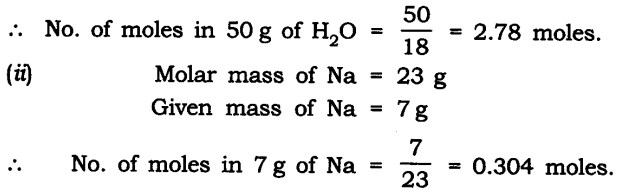Ques 10: Find the number of atoms in the following:
(i) 0.5 mole of C atom
(ii) 2 mole of N atom
Ans: (i) 0.5 mole of C atom:
Number of atoms in 1 mole of C atom = 6.022 x 1023 atoms
Number of atoms in 0.5 mole of C atom = 6.022 x 1023 x 0.5
= 3.011 x 1023 atoms
(ii) 2 mole of N atom:
Number of atoms in 1 mole of N atom = 6.022 x 1023 atoms
Number of atoms in 2 mole of N atom = 6.022 x 2 x 1023
= 1.2044 x 1024 atoms

Ques 11: Find the mass of the following:
(i) 6.022 x 1023 number of O2 molecules
(ii) 1.5 mole of CO2 molecule
Ans: (i) 6.022 x 1023 number of 02 molecules:
Mass of 1 mole of Omolecule = 6.022 x 1023 molecules = 32 g
(ii) 1.5 mole of CO2 molecule:
Mass of 1 mole of C02 molecule = 6.022 x 1023 molecules = 44 g
Mass of 1.5 mole C02 molecule = 44 x 1.5 = 66 g

Ques 12: Show the relationship between mole, Avogadro number and mass.
Ans: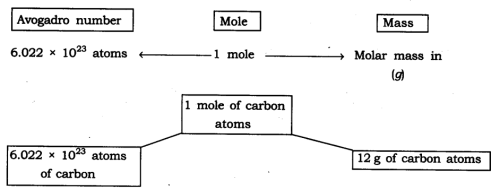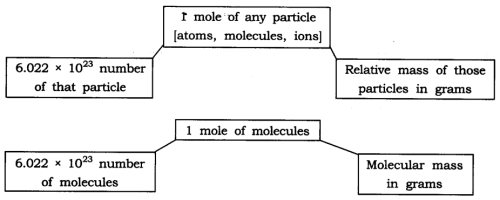Ques 13: What are the rules for writing the symbol of an element?
Ans: IUPAC —> International Union of Pure and Applied Chemistry approves name of elements.
Symbols are the first one or two letters of the element’s name in English. The first letter of a symbol is always written as a capital letter (upper case) and the second letter as a small letter (lower case).
e.g., Hydrogen —> H Helium —> He
Some symbols are taken from the names of elements in Latin, German or Greek.
e.g., Symbol of iron is Fe, its Latin name is Ferrum.
Symbol of sodium is Na, its Latin name is Natrium.

Ques 14: Explain relative atomic mass and relative molecular mass.
Ans: Relative atomic mass: It can be defined as the number of times one atom of given element is heavier than 1/12 th of the mass of an atom of carbon-12. Relative Molecular Mass: It is defined as the number of times one molecule
of a substance or given element is heavier than 1/12 th of the mass of one atom of carbon-12.

Ques 15: The formula of carbon-dioxide is CO2. What information do you get from this formula?
Ans: (i) CO2 represents carbon-dioxide.
(ii) CO2 is one molecule of carbon-dioxide.
(iii) CO2 is one mole of carbon-dioxide i.e., it contains 6.022 x 1023 molecules of carbon dioxide.
(iv) CO2 contains 1 atom of carbon and two atoms of oxygen.
(v) CO2 represents 44 g of molar mass.

Ques 16: State 3 points of difference between an atom and an ion.
Ans:

 Atom Ion An atom has no charge. An ion has either positive or negative charge. Number of electrons = number of protons. Number of electrons ≠ number of protons. Atom is reactive Ion is stable

Ques 17: Calculate the formula unit mass of NaCl and CaCl2.
(Na = 23, Cl = 35.5, Ca = 40)
Ans:  Formula unit mass of NaCl = 23 + 35.5
= 58.5 u
Formula unit mass of CaCl2 = 40 + (2 x 35.5)
= 40 + 71 = 111 u

Ques 18: The ratio by mass for hydrogen and oxygen in water is given as 1 : 8 respectively. Calculate the ratio by number of atoms for a water molecule.
Ans: The ratio by number of atoms for a water molecule are:

 Element Ratio by mass Atomic mass Mass ratio/Atomic mass Simplest ratio H 1 1 1/1 = 1 2 O 8 16 8/16 = 1/2 1

Thus, the ratio by number of atoms for water is H : O = 2 : 1.

Ques 19: Write down the chemical formula for the following compounds:
(a) Aluminium carbonate
(b) Calcium sulphide
(c) Zinc carbonate
(d) Copper phosphate
(e) Magnesium bicarbonate
(f) Aluminium hydroxide.
Ans: The chemical formula are: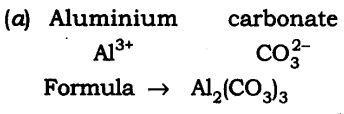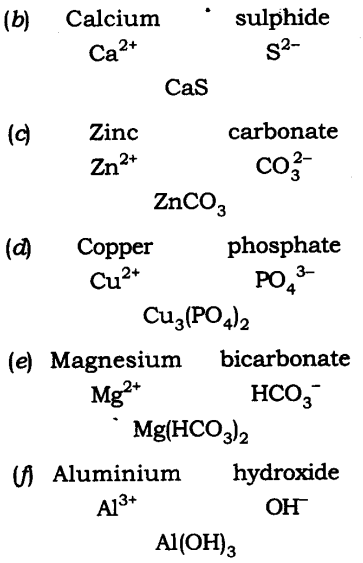Ques 20: Give the atomicity of the following compounds:
(a) Ca(OH)2 (d) H2S04
(b) Mg(HC03)2 (e) Al2(S04)3
(c) Cu20. (f) MgCl2
Ans: The atomicity of the molecules are: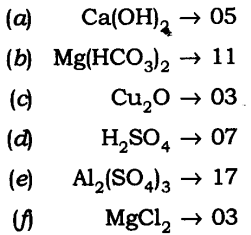Ques 21: Explain the difference between 20, 02 and 03.
Ans: 2O —> It represents 2 atoms of oxygen (cannot exist independently).
O2 —> It represents one molecule of oxygen (made up of 2 atom) can exist freely.
O3 —> It represents one molecule of ozone (made up of 3 atoms) it can exist independently.

Ques 1: (a) How do atoms exist?
(b) What is atomicity?
(c) What are polyatomic ions?
Ans: (a) Atoms of some elements are not able to exist independently. For such elements atoms form molecules and ions. In case of metals and inert gases atoms can exist independently.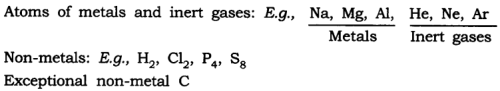(b) The number of atoms constituting a molecule is known as its atomicity.
E.g.,O3 —> atomicity is 3
O2 —> atomicity is 2
(c) Polyatomic ions: When more than two atoms combine together and act like an atom with a charge on it is called polyatomic ion.
E.g., OH, N03, NH4+

Ques 2: Calculate
(a) the mass of one atom of oxygen
(b) the mass of one molecule of oxygen
(c) the mass of one mole of oxygen gas
(d) the mass of one ion of oxygen
(e) the number of atoms in 1 mole of oxygen molecule
Ans: (a) Mass of one atom of oxygen
1 mole of oxygen atom  = 16 gm = 6.022 x 1023 atoms.
∴ Mass of one atom of oxygen = 16/6.022 x 1023 = 2.65 x 1023
(b) Mass of one molecule of oxygen
1 molecule of oxygen = O2
= 2 x 16
= 32 u
(c) Mass of oneoxygen gas
1 molecule of oxygen gas is O2 = 32 u
(d) Mass of one ion of oxygen
One mole of oxygen = 6.022 x 1023 atoms = 16g. 16
Mass of one ion of oxygen =  16/6.022 x 1023
= 2.65 x 1023
(e) Number of atoms in one mole of oxygen molecule 1 mole of oxygen molecule i.e.
0= 6.022 x 1023 molecules.
1 molecule of 02 = 2 atoms.
∴ Number of atoms in 1 mole of oxygen molecule = 6.022 x 1023 x 2 atoms
= 1.2044 x 1024 atoms

Ques 3: What is meant by atomic mass, gram atomic mass of an element? Why is the mass have different expressions i.e., ‘u’ and ‘g’?
Ans: The atoms are very tiny and their individual mass cannot be calculated as it is negligible. Hence the mass of atoms is expressed in units with respect to a fixed standard. Initially hydrogen atom with mass 1 was taken as standard unit by Dalton. Later, it was replaced by oxygen atom (0=16). But due to the isotopes the masses were found in fractions instead of whole number. Hence, carbon (C=12) isotope was taken as standard unit and was universally accepted.
The atomic mass unit is equal to one twelfth (1/12) the mass of an atom of carbon-12, its unit is u.
Gramatomic mass: When the atomic mass of an element is expressed in grams, it is called the gramatomic mass of the element.
The mass of atoms, molecules is expressed in ‘u’ and the mass of moles i.e., molar mass is expressed in g.

Ques 4: Define a mole. Give the significance of the mole.
Ans: Mole-One mole of any species (atoms, molecules, ions or particles) is that quantity or number having a mass equal to its atomic or molecular mass in grams.
1 mole = 6.022 x 1023 in number (atoms, molecules, ions or particles)
Significance of the mole
1. A mole gives the number of entities present i.e, 6.022 x 1023 particles of the substance.
2. Mass of 1 mole is expressed as M grams.
3. Mass of 1 mole = mass of 6.022 x 1023 atoms of the element.
E.g., 1 mole of O2 = 6.022 x 1023 atoms
2 x 16 = 32 g
6.022 x 2 x 1023 = 1.2044 x 104 atoms
1 mole of (compound) HCl = 6.022 x x 1023 atoms of H and Cl atoms
(1 + 35.5 = 36.5 g)   (6.022 x 1023 molecules of HC1)

Offer running on EduRev: Apply code STAYHOME200 to get INR 200 off on our premium plan EduRev Infinity!

,

,

,

,

,

,

,

,

,

,

,

,

,

,

,

,

,

,

,

,

,

;